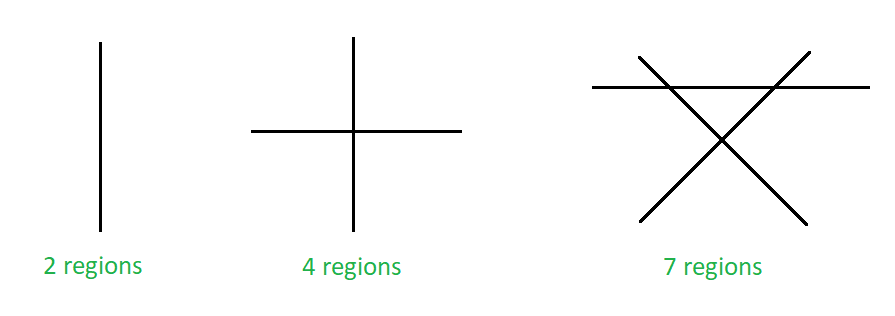# Maximum number of region in which N non-parallel lines can divide a plane

Given N‘, the number of non-parallel lines. The task is to find the maximum number of regions in which these lines can divide a plane.

Examples:

Input : N = 3
Output : 7

Input : N = 2
Output : 4

## Recommended: Please try your approach on {IDE} first, before moving on to the solution.Maximum number of regions on a plane formed due to non-parallel lines

Approach : The above image shows the maximum number of regions a line can divide a plane. One line can divide a plane into two regions, two non-parallel lines can divide a plane into 4 regions and three non-parallel lines can divide into 7 regions and so on. When the nth line is added to a cluster of (n-1) lines then the maximum number of extra regions formed is equal to n.

Now solve the recursion as follows:

L(2) – L(1) = 2 … (i)
L(3) – L(2) = 3 … (ii)
L(4) – L(3) = 4 … (iii)
. . .
. . .
L(n) – L(n-1) = n ; … (n)
Adding all the above equation we get,

L(n) – L(1) = 2 + 3 + 4 + 5 + 6 + 7 + …… + n ;
L(n) = L(1) + 2 + 3 + 4 + 5 + 6 + 7 + …… + n ;
L(n) = 2 + 2 + 3 + 4 + 5 + 6 + 7 + …… + n ;
L(n) = 1 + 2 + 3 + 4 + 5 + 6 + 7 + …… + n + 1 ;
L(n) = n ( n + 1 ) / 2 + 1 ;

The number of region in which N non-parallel lines can divide a plane is equal to N*( N + 1 )/2 + 1.

Below is the implementation of the above approach:

## C++

 `// C++ program to implement the above problem ` ` `  `#include ` `using` `namespace` `std; ` ` `  `// Function to find the maximum ` `// number of regions on a plane ` `void` `maxRegions(``int` `n) ` `{ ` `    ``int` `num; ` `    ``num = n * (n + 1) / 2 + 1; ` ` `  `    ``// print the maximum number of regions ` `    ``cout << num; ` `} ` ` `  `// Driver code ` `int` `main() ` `{ ` `    ``int` `n = 10; ` ` `  `    ``maxRegions(n); ` ` `  `    ``return` `0; ` `} `

## Java

 `// Java program to implement the above problem ` `class` `GFG ` `{ ` ` `  `    ``// Function to find the maximum ` `    ``// number of regions on a plane ` `    ``static` `void` `maxRegions(``int` `n)  ` `    ``{ ` `        ``int` `num; ` `        ``num = n * (n + ``1``) / ``2` `+ ``1``; ` ` `  `        ``// print the maximum number of regions ` `        ``System.out.println(num);; ` `    ``} ` ` `  `    ``// Driver code ` `    ``public` `static` `void` `main(String[] args)  ` `    ``{ ` `        ``int` `n = ``10``; ` `        ``maxRegions(n); ` `    ``} ` `} ` ` `  `// This code is contributed by 29AjayKumar `

## Python3

 `# Python3 program to implement ` `# the above problem ` ` `  `# Function to find the maximum ` `# number of regions on a plane ` `def` `maxRegions(n): ` `    ``num ``=` `n ``*` `(n ``+` `1``) ``/``/` `2` `+` `1` ` `  `    ``# print the maximum number ` `    ``# of regions ` `    ``print``(num) ` ` `  `# Driver code ` `n ``=` `10` ` `  `maxRegions(n) ` ` `  `# This code is contributed ` `# by Mohit Kumar `

## C#

 `// C# program to implement the above problem ` `using` `System; ` `     `  `class` `GFG ` `{ ` ` `  `    ``// Function to find the maximum ` `    ``// number of regions on a plane ` `    ``static` `void` `maxRegions(``int` `n)  ` `    ``{ ` `        ``int` `num; ` `        ``num = n * (n + 1) / 2 + 1; ` ` `  `        ``// print the maximum number of regions ` `        ``Console.WriteLine(num); ` `    ``} ` ` `  `    ``// Driver code ` `    ``public` `static` `void` `Main(String[] args)  ` `    ``{ ` `        ``int` `n = 10; ` `        ``maxRegions(n); ` `    ``} ` `} ` ` `  `// This code is contributed by 29AjayKumar `

Output:

```56
```

Time Complexity : O(1)

Attention reader! Don’t stop learning now. Get hold of all the important DSA concepts with the DSA Self Paced Course at a student-friendly price and become industry ready.

My Personal Notes arrow_drop_upCheck out this Author's contributed articles.

If you like GeeksforGeeks and would like to contribute, you can also write an article using contribute.geeksforgeeks.org or mail your article to contribute@geeksforgeeks.org. See your article appearing on the GeeksforGeeks main page and help other Geeks.

Please Improve this article if you find anything incorrect by clicking on the "Improve Article" button below.